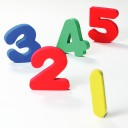LessonsSay "Hello" in Turkish Anytime, Anywhere!
Absolute Beginner Season 1
Learn how to greet people and use personal suffixes

Start Learning Turkish in the next 30 Seconds with
a Free Lifetime AccountNumbers
30 wordsNumbers
30 words
sıfır
(n)
zero
sıfır numara
number zero
bir
(n)
one
bir derece
one degree
iki
(n)
two
İki benim favori sayım.
The number two is my favorite number.
üç
(n)
three
üç derece
three degrees
dört
(n)
four
dört derece
four degrees
beş
(n)
five
beş derece
five degrees
altı
(n)
six
altı derece
six degrees
yedi
(n)
seven
yedi şey
seven things
sekiz
(n)
eight
sekiz derece
eight degrees
dokuz
(n)
nine
dokuz derece
nine degrees
on
(n)
ten
on derece
ten degrees
yirmi bir
(n)
twenty-one
Yirmi bir numara
number twenty-one
yirmi iki
(n)
twenty-two
yirmi iki numara
number twenty-two
otuz üç
(n)
thirty-three
Otuz üç numara
number thirty-three
kırk dört
(n)
forty-four
Kırk dört numara
number forty-four
elli beş
(n)
fifty-five
Elli beş numara
number fifty-five
altmış altı
(n)
sixty-six
altmış altı numara
number sixty-six
yetmiş yedi
(n)
seventy-seven
Yetmiş yedi numara
number seventy-seven
seksen sekiz
(n)
eighty-eight
seksen sekiz numara
number eighty-eight
doksan dokuz
(n)
ninety-nine
yüzde doksan dokuz
ninety-nine percent
yüz
(n)
one hundred
Yüz numara
number one hundred
bin
(n)
one thousand
Bin sayfa
one-thousand pages
iki bin
(n)
two thousand
O iki bin dolar değerinde.
It costs two thousand dollars.
üç bin
(n)
three thousand
dört bin
(n)
four thousand
Dört bin dört yüz kırk
four thousand four hundred and forty